### Home > PC > Chapter 7 > Lesson 7.3.1 > Problem7-95

7-95.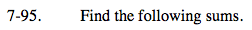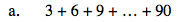Add the first and last terms.
Divide by 2.
Multiply by the number of terms.

$\frac{30(3+90)}{2}=1395$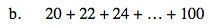How many terms are there?

41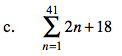Add the first and last terms.
Divide by 2.
Multiply by the number of terms.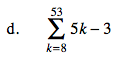How many terms are there? That is, if the sum started with k = 1, what would it end with?

46